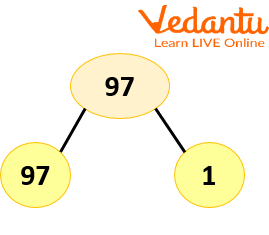Courses
Courses for Kids
Free study material
Offline Centres
More

# Factors of 97Last updated date: 05th Dec 2023
Total views: 59.7k
Views today: 0.59k## Factors of 97: An Introduction

Factors are a part of our daily life. We can use them in daily life scenarios such as arranging items in a box, handling money, finding patterns in numbers, solving ratios and working with expanding or reducing fractions. A number has both positive and negative factors. For example, let's determine the factors of 8. Since the number 8 is divisible by 1, 2, 4, and 8, we can list these as the positive factors of 8. The product of two negative numbers is a positive number, hence $(-1)\times (-8) = 8$ and $(-2)\times (-4) = 8.$

In addition, the number 8 has negative elements, which can be written as -1, -2, -4, and -8. However, we generally use the positive factors of a number. To find the factors of 97, we have to find the numbers which divide 97 completely, leaving no remainders. The factors of 97 are the numbers which exactly divide 97. Since 97 is a prime number, it has only 2 factors 1 and 97.

## What are the factors of 97?

To calculate factors of 97, we need to find all the numbers that divide 97 without leaving any remainder. We start with the number 1 and then check 2, 3, 4, and 5 up to 97, respectively. The number 1 and the number itself would always be a factor of any given number. If we check the list of prime numbers, we can see that 97 is the 25th prime number. Thus, it has only 2 factors, 1 and 97. Let us check if 97 has any other factors by dividing it by other integers.

The table below shows the quotient obtained when each integer is divided by 97.

 Numbers which divides 97 Quotient 1 97 2 48.5 3 32.33 4 24.5 5 19.4 6 16.1667 7 13.8571 8 12.125 9 10.7778

## Division Method to Find the Factors of 97

Finding prime factors of 97 using the division method

Step 1: We first start dividing 97 by the smallest prime number ie 2. But it is not divisible by 2.

Step 2 : We then move on to the next prime number 3, but it also not divisible by 3. We then divide it by 5, 7, 11 , 13…. but 97 is not divisible by any of them.

Step 3: After finding the smallest prime factor of 97 ie 97, divide 97 by 97 to get 1 as the quotient.

## Factor Pairs of 97

Factor pairs or pair factors of a number are factors of a number given in pairs which, when multiplied together, give the original number. They can be only whole numbers and integers. The smallest factor of a number is 1, and the biggest factor of a number is the number itself.

 Positive factor pair Negative factor pair Paired factorisation 1 and 97 −1 and −97 1×97 = 97

Factors Tree of 97Factors tree of 97

## Prime Factors of 97

97 have two factors 1 and 97; 1 is neither prime nor composite. This is because 97 is a prime number. Therefore, it is the only prime factor of 97.

## Properties of Factors of a Number

The qualities of a number's factors are as follows:

• A number has a finite number of factors.

• A number's prime factors will never be more than the provided number.

• Every number contains at least two factors, 1 and the actual number, with the exception of 0 and 1.

• Finding a number's factors involves using division and multiplication operations.

## Key Features

• To find the factors of 97, we divide it by the numbers from 1 to 97 and check which are the numbers which divide it without leaving any remainder.

• We use the division method, factor pairs and factor tree to find the factors of 97.

• 97 is a prime number, and its only prime factor is 97.

## Solved Examples

1. Find the sum of all the factors of 97.

Ans: We know that the factors of 97 are 1 and 97.

Therefore, the sum of the factors is 1 + 97 = 98.

2. What are the common factors of 97, 71 and 83.

Ans: Factors of 97 = 1 and 97

Factors of 71 = 1 and 71

Factors of 83 = 1 and 83

Since 97, 71 and 83 are prime numbers, 1 is the only common factor.

## FAQs on Factors of 97

1. What are factor pairs?

A factor pair is a set of two factors, which when multiplied together, give a that number. In other words, two numbers are multiplied to get a product and these two terms’ product is a factor pair of the result. For example, 2 and 3 are multiplied to get 6. So 2 and 3 are the factor pairs of 6.

2. What is a prime and a composite number?

There are numbers which have only 2 factors, 1 and the number itself. They are called prime numbers. Composite numbers have more than 2 factors.

3. Is 1 a prime or a composite number?

1 has only 1 factor ie 1. So it is not prime as prime numbers have 2 factors, and neither is it composite as composite numbers have more than 2 factors.

4. How many factors does 97 have?

Since 97 is a prime number it has only two factors, 1 and 97.